# R语言基于递归神经网络RNN的温度时间序列预测

• _删除_层/每层的单位数(模型) 如L1或L2正则化所述,过度复杂的模型更有可能过度_拟合_，可以使用删除来抵抗重复图层的过拟合。
• 堆叠循环层 —这增加了网络的表示能力（以更高的计算负荷为代价）。
• 双向循环层 —这些_层_以不同的方式向循环网络提供相同的信息，从而提高准确性。

# 温度预测问题

`````` unzip(
"climate.csv.zip",
)``````

LSTM神经网络架构和原理及其在Python中的预测应用

``````library(tibble)

glimpse(data)``````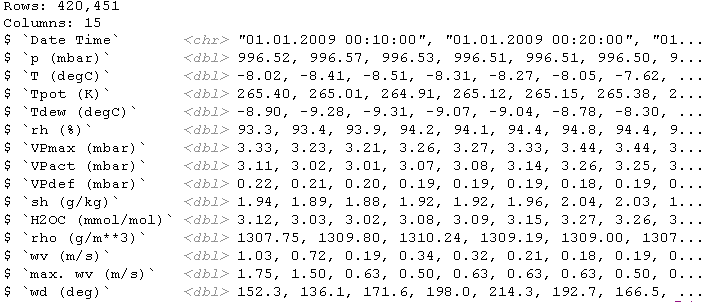`` ggplot(data, aes(x = 1:nrow(data), y = `degC`)) + geom_line()``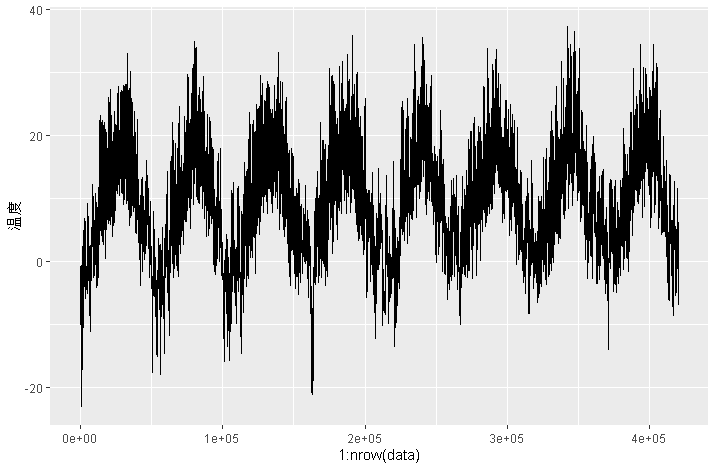# R语言神经网络模型预测车辆数量时间序列

``ggplot(data[1:1440,], aes(y = `degC`)) + geom_line()``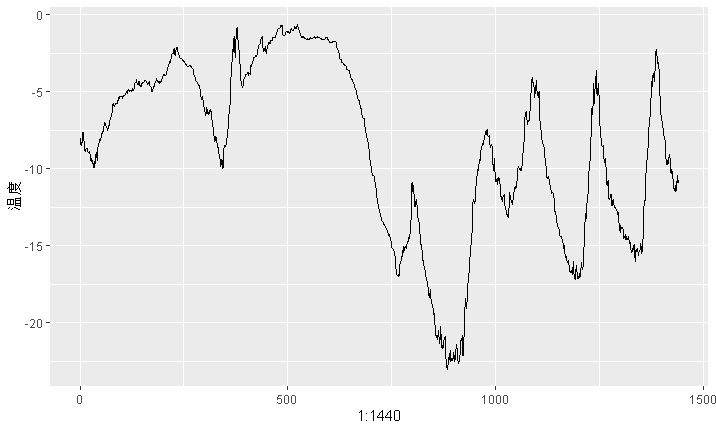# 准备数据

• `lookback = 1440` —观察将追溯到10天。
• `steps = 6` —观测将在每小时一个数据点进行采样。
• `delay = 144` —目标将是未来的24小时。

• 将数据预处理为神经网络可以使用格式。数据已经是数字了，因此您无需进行任何向量化。但是数据中的每个时间序列的度量尺度都不同（例如，温度通常在-20至+30之间，但以毫巴为单位的大气压约为1,000）。您将独立地标准化每个时间序列。
• 编写一个生成器函数，该函数将获取当前的浮点数据数组，并生成来自最近的过去以及将来的目标温度的成批数据。由于数据集中的样本是高度冗余的（样本 N 和样本 N  + 1将具有大多数相同的时间步长），因此显式分配每个样本会很浪费。相反，您将使用原始数据即时生成样本。

`````` gen <- sequence_generator(10)
gen()``````
`` 10``
``gen()``
`` 11``

# 生成器的当前状态`value` 是在函数外部定义的 变量。superassignment（`<<-`）用于从函数内部更新此状态。

``data <- data.matrix(data[,-1])``

``````train_data <- data[1:200000,]
data <- scale(data, center = mean, scale = std)``````

• `data` —原始的浮点数据数组。
• `lookback` —是_输入数据应该_包括多少个_时间_步。
• `delay` —目标应该在未来多少步。
• `min_index` 和 `max_index` —`data` 数组中的索引， 用于定义从中提取时间步长。保留一部分数据用于验证和另一部分用于测试。
• `shuffle` —随机整理样本还是按时间顺序绘制样本。
• `batch_size` —每批样品数。
• `step` —采样数据的时间段（以时间为单位）。您将其设置为6，以便每小时绘制一个数据点。

``````lookback <- 1440
step <- 6

# 为了查看整个验证集，需要从valu gen中提取多少步骤
val_steps <- (300000 - 200001 - lookback) / batch_size

# 为了查看整个测试集，需要从testu gen中提取多少步骤
test_steps <- (nrow(data) - 300001 - lookback) / batch_size``````

# 常识性的非机器学习基准

``mean(abs(preds - targets))``

`````` for (step in 1:val_steps) {

preds <- samples[,dim(samples)[],2]
mae <- mean(abs(preds - targets))
batch_maes <- c(batch_maes, mae)
}
print(mean(batch_maes)) ``````

MAE为0.29。由于温度数据已标准化为以0为中心并且标准偏差为1。它的平均绝对误差为0.29 x `temperature_std` 摄氏度：2.57˚C。

``celsius_mae <- 0.29 * std[]``

# 基本的机器学习方法

``````model_sequential() %>%
layer_flatten(input_shape = c(lookback / step, dim(data)[-1])) %>%

history <- model %>% fit_generator(
train_gen,
steps_per_epoch = 500,
epochs = 20,
validation_data = val_gen,
validation_steps = val_steps
)``````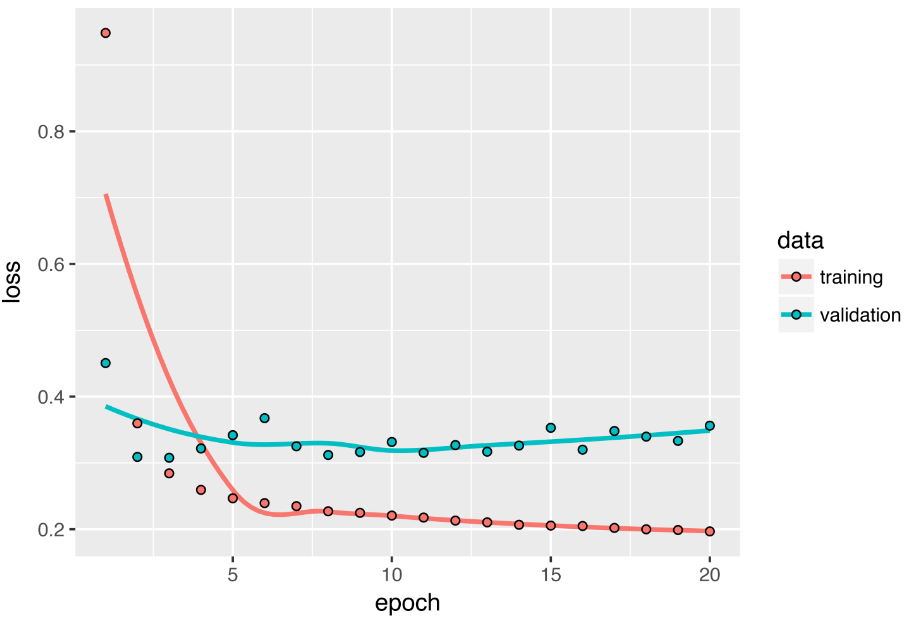# 基准模型

``````model_sequential() %>%
layer_gru(units = 32, input_shape = list(NULL, dim(data)[[-1]])) %>%
layer_dense(units = 1)

model %>% fit_generator(
train_gen,
steps_per_epoch = 500,
epochs = 20, ``````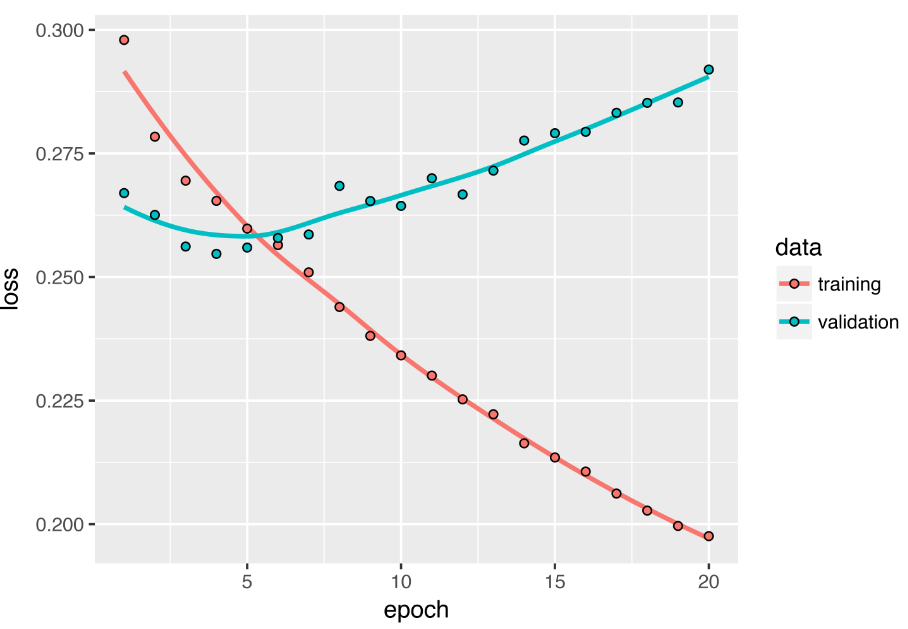# 丢弃（dropout）对抗过度拟合

Yarin Gal使用Keras进行了研究，并帮助将这种模型直接构建到Keras循环层中。Keras中的每个循环图层都有两个与dropout相关的参数：  `dropout`，一个浮点数，用于指定图层输入单元的dropout率；以及 `recurrent_dropout`，用于指定循环单元的dropout率。由于使用丢失dropout进行正则化的网络始终需要更长的时间才能完全收敛，因此您需要两倍的时间训练网络。

``````model_sequential() %>%
layer_gru(units = 32, dropout = 0.2, recurrent_dropout = 0.2, ``````

# 下图显示了结果。在前20个时期中，您不再过度拟合。但是，尽管您的评估分数较为稳定，但您的最佳分数并没有比以前低很多。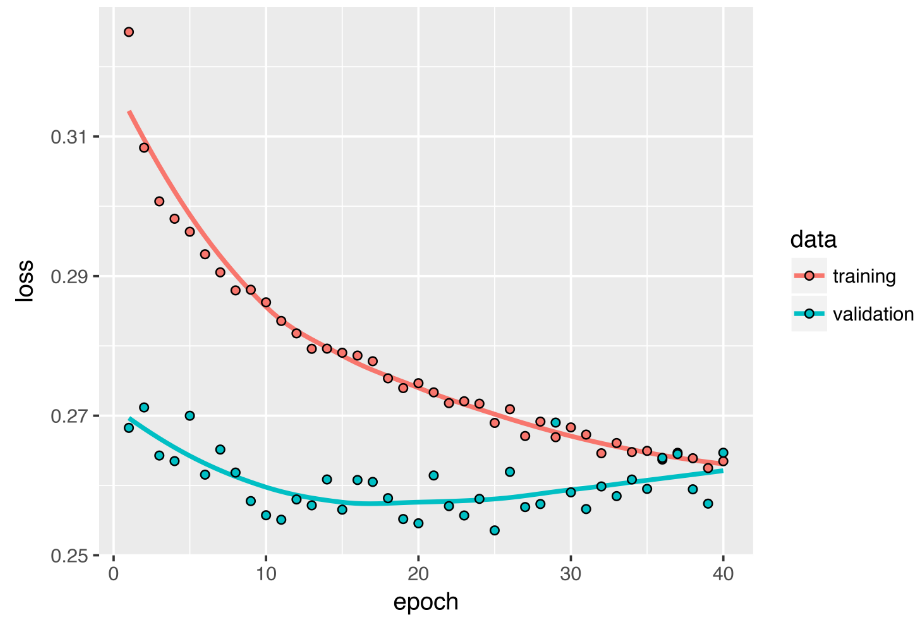# 堆叠循环图层

``````model_sequential() %>%
layer_gru(units = 32,
dropout = 0.1,
recurrent_dropout = 0.5,
return_sequences = TRUE,
input_shape = list(NULL, dim(data)[[-1]])) %>%
layer_gru(units = 64, activation = "relu",
dropout = 0.1,
recurrent_dropout = 0.5) %>% ``````

• 因为不需要过度拟合的问题，所以可以安全地增加图层大小以寻求验证损失的改善。但是，这具有不可忽略的计算成本。
• 添加层并没有很大的帮助，因此此时您可能会看到网络容量增加带来的收益递减。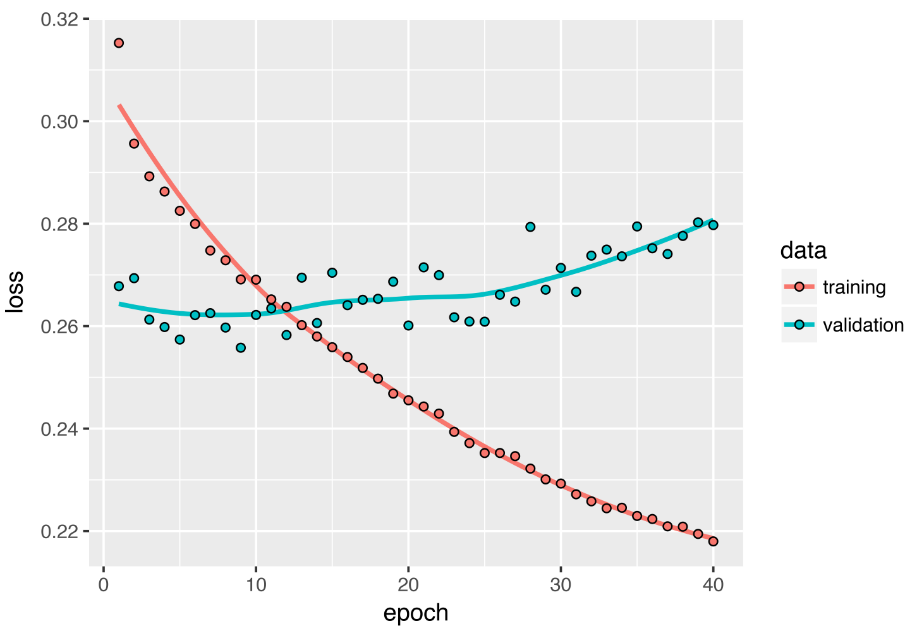# 使用双向RNN

RNN特别依赖于顺序或时间的：它们按顺序处理输入序列的时间步长，重新排列时间步长可以完全改变RNN从序列中提取的表示形式。

# 本节介绍的最后一种技术称为 _双向RNN_。双向RNN是常见的RNN变体，在某些任务上可以提供比常规RNN更高的性能。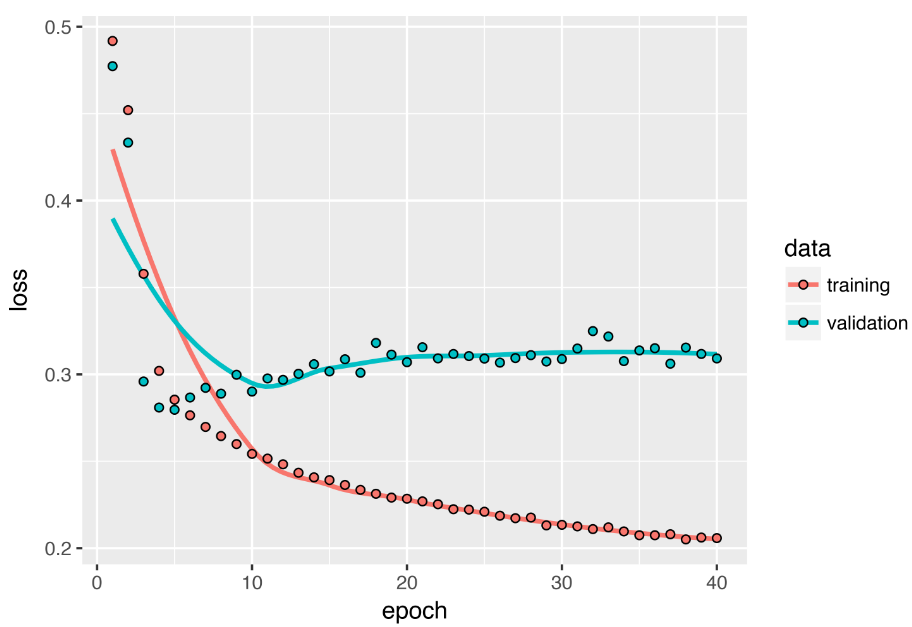``````# 作为特征考虑的单词数量
max_features <- 10000

c(c(x_train, y_train), c(x_test, y_test)) %<-% imdb

# 反转序列
x_train <- lapply(x_train, rev)
x_test <- lapply(x_test, rev)

model <- keras_model_sequential() %>%
layer_embedding(input_dim = max_features, output_dim = 128) %>%
layer_lstm(units = 32) %>% ``````

# 双向RNN利用此思想来改进按时间顺序排列的RNN的性能。

``````model <- keras_model_sequential() %>%
layer_embedding(input_dim = max_features, output_dim = 32) %>%
bidirectional(
layer_lstm(units = 32)

model %>% compile(
optimizer = "rmsprop",
loss = "binary_crossentropy", ``````

``````model_sequential() %>%
bidirectional(
layer_gru(units = 32), input_shape = list(NULL, dim(data)[[-1]])

model %>% fit_generator(
train_gen,
steps_per_epoch = 500,
epochs = 40, ``````

# 更进一步

• 调整堆叠设置中每个循环图层的单位数。
• 调整`RMSprop` 优化器使用的学习率 。
• 尝试使用 `layer_lstm` 代替 `layer_gru`
• 尝试在循环层的顶部使用更大的紧密连接的回归变量：即，更大的密集层，甚至一叠密集层。
• 不要忘记最终在测试集上运行性能最佳的模型（就验证MAE而言），否则，您将开发过度拟合验证集的结构。

# 我们可以提供一些准则，建议在给定问题上可能起作用或不起作用的因素，但是最终，每个问题都是唯一的；

Kaizong Ye拓端研究室（TRL）的研究员。

​非常感谢您阅读本文，如需帮助请联系我们！QQ在线咨询

15121130882

0571-63341498

## 关注有关新文章的微信公众号

This will close in 0 seconds